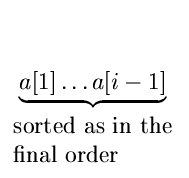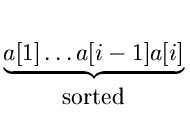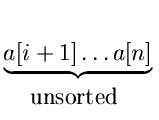Next:9.2 Insertion SortUp:9. Sorting MethodsPrevious:9. Sorting Methods

# 9.1 Bubble Sort

Let a, a,..., a[n] be n records to be sorted on a key field key''. In bubble sort, records with low keys (light records) bubble up to the top.

{

for(i = 1;i < = n - 1;i + +)

{

for(j = n;j > = i + 1;j - -)

if (a[j].key <a[j - 1].key)

swap the records a[j] and a[j - 1]

}

}

At the end of the (i - 1)st iteration, we haveIn the ith iteration, the ith lowest key bubbles up to the ithposition and we getWorst case complexity and also average case complexity is O(n2).

eEL,CSA_Dept,IISc,Bangalore